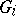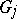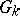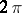# Glueing method

(diff) ← Older revision | Latest revision (diff) | Newer revision → (diff)

in the theory of surfaces

A method for constructing surfaces isometric to a given one. The glueing method has applications in proving the realizability of abstractly-defined convex metrics, in questions of bending convex surfaces, and in quantitative estimates of bendability. Its strength lies in the fact that it works in situations where differential equations are powerless.

Aleksandrov's theorem: Letbe closed domains in a manifold with an intrinsic metric of positive curvature, bounded by a finite number of curves with bounded rotational variation. Letbe the manifold consisting of the domainswith their boundaries identified in such a way that:

1) the identified segments of the boundaries ofandhave equal length;

2) the sum of the rotations of the identified segments of the boundaries ofandfrom the sides of these domains is non-negative;

3) the sum of the angles made by the sectors at the identified points of the domainswith the sides of these domains does not exceed.

Thenhas an intrinsic metric of positive curvature that coincides with the metrics of the domains in neighbourhoods of corresponding points.

How to Cite This Entry:
Glueing method. Encyclopedia of Mathematics. URL: http://encyclopediaofmath.org/index.php?title=Glueing_method&oldid=18059
This article was adapted from an original article by M.I. Voitsekhovskii (originator), which appeared in Encyclopedia of Mathematics - ISBN 1402006098. See original article